Question

# A box contains 6 red balls, 4 yellow balls, and 10 white balls. The experiment consists...

A box contains 6 red balls, 4 yellow balls, and 10 white balls. The experiment consists of taking three balls from the box, without replacement, and seeing how many of each color you took. What is the probability that exactly 1 balls will be white? Calculate your answer rounded to three decimal places.

Here we are given we have 6 red 4 yellow and 10 white balls then we are to find probability that exactly one is white

=10c1*10c2/20c3=0.395

#### Earn Coins

Coins can be redeemed for fabulous gifts.

Similar Homework Help Questions
• ### (5) A box contains 4 red and 6 white balls. If two balls are drawn at...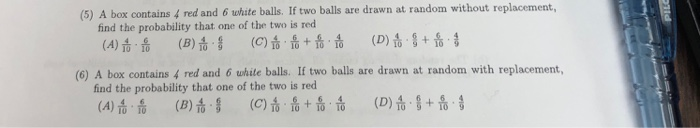(5) A box contains 4 red and 6 white balls. If two balls are drawn at random without replacement, find the probability that one of the two is red (6) A box contains 4 red and 6 white balls. If two balls are drawn at random with replacement, find the probability that one of the two is red

• ### Problems 9 and 10 A box contains 2 red balls, 3 white and 1 blue balls....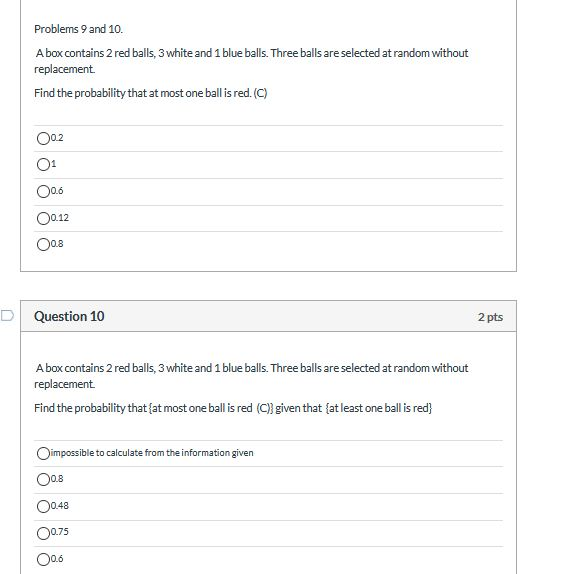Problems 9 and 10 A box contains 2 red balls, 3 white and 1 blue balls. Three balls are selected at random without replacement Find the probability that at most one ballis red. (C) 00.2 01 O 0.6 0.12 0.8 Question 10 2 pts A box contains 2 red balls, 3 white and 1 blue balls. Three balls are selected at random without replacement Find the probability that {at most one ball is red (C) given that at least one...

• ### 6. A box contains 30 red balls, 30 white balls, and 30 blue balls. If 10 balls are selected at random, without repla...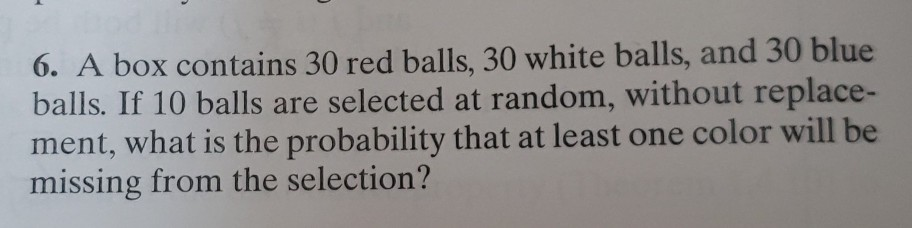6. A box contains 30 red balls, 30 white balls, and 30 blue balls. If 10 balls are selected at random, without replace- ment, what is the probability that at least one color will be missing from the selection? 6. A box contains 30 red balls, 30 white balls, and 30 blue balls. If 10 balls are selected at random, without replace- ment, what is the probability that at least one color will be missing from the selection?

• ### Refer to Example 4.40. An urn contains six red balls, six white balls, and six blue...

Refer to Example 4.40. An urn contains six red balls, six white balls, and six blue balls, and sample of four balls is drawn at random without replacement. Compute the probability that all of the balls in the sample are the same color. (Round your answer to four decimal places.) b) An urn contains eight red balls, eight white balls, and eight blue balls, and sample of five balls is drawn at random without replacement. Compute the probability that the...

• ### (+5)* 6. Suppose that a box contains 2 red balls, 3 white balls, and 4 blue...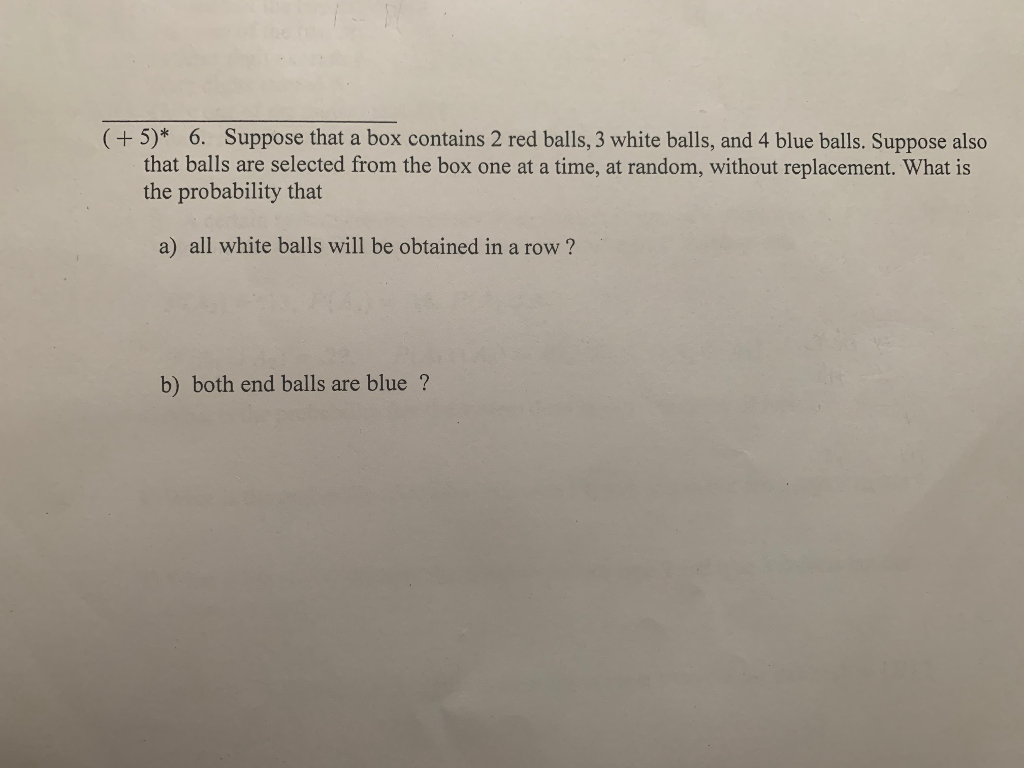(+5)* 6. Suppose that a box contains 2 red balls, 3 white balls, and 4 blue balls. Suppose also that balls are selected from the box one at a time, at random, without replacement. What is the probability that a) all white balls will be obtained in a row? b) both end balls are blue?

• ### 4. *A box contains 3 white balls, 4 black balls, and 3 red balls. Consider selecting...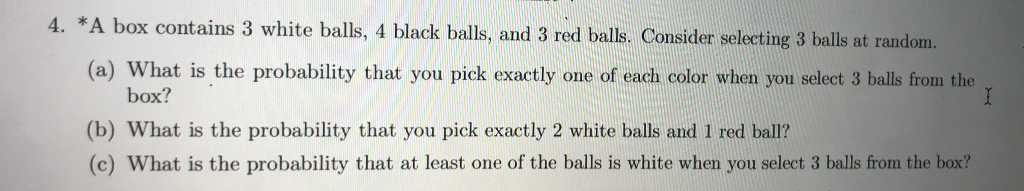4. *A box contains 3 white balls, 4 black balls, and 3 red balls. Consider selecting 3 balls at random (a) What is the probability that you pick exactly one of each color when you select 3 balls from the box? (b) What is the probability that you pick exactly 2 white balls and 1 red ball? (c) What is the probability that at least one of the balls is white when you select 3 balls from the box?

• ### Probability question *A box contains 3 white balls, 4 black balls, and 3 red balls. Consider...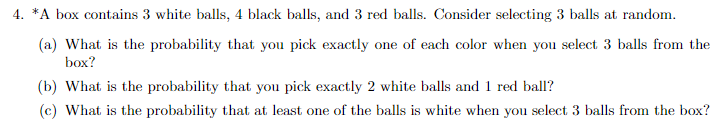Probability question *A box contains 3 white balls, 4 black balls, and 3 red balls. Consider selecting 3 balls at random. (a) What is the probability that you pick exactly one of each color when you select 3 balls from the box? (b) What is the probability that you pick exactly 2 white balls and 1 red ball? (c) What is the probability that at least one of the balls is white when you select 3 balls from the box?

• ### A box contains one yellow, two red, and three green balls. Two balls are randomly chosen...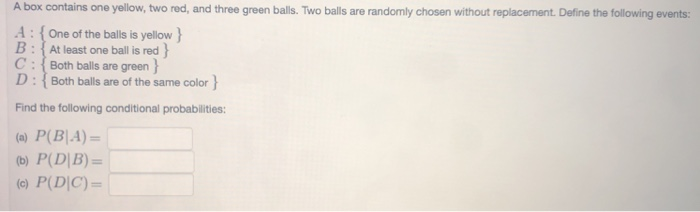A box contains one yellow, two red, and three green balls. Two balls are randomly chosen without replacement. Define the following events A: One of the balls is yellow B: At least one ball is red h C: Both balls are green D:Both balls are of the same color ) Find the following conditional probabilities: (a) P(BA)- b) P(DB)- (c) P(DIC)-

• ### lomework Assignments (1 point) A box contains one yellow, two red, and three green balls. Two balls are randomly chosen...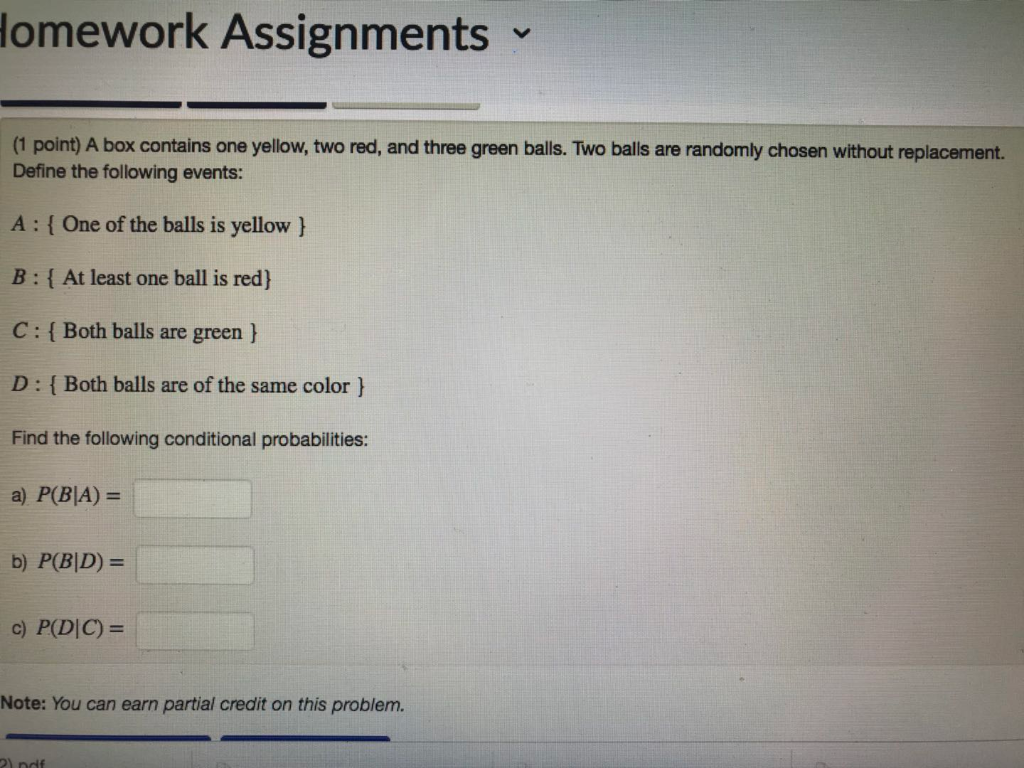lomework Assignments (1 point) A box contains one yellow, two red, and three green balls. Two balls are randomly chosen without replacement. Define the following events: A: One of the balls is yellow B: At least one ball is red) C: i Both balls are green h D: I Both balls are of the same color Find the following conditional probabilities: a) P(BIA) b) P(BD)- Note: You can earn partial credit on this problem. lomework Assignments (1 point) A box...

• ### 2. A box contains 5 red balls, 7 white balls, and 8 black balls. Four balls...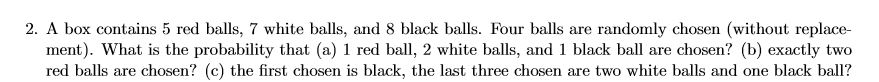2. A box contains 5 red balls, 7 white balls, and 8 black balls. Four balls are randomly chosen (without replace- ment). What is the probability that (a) 1 red ball, 2 white balls, and 1 black ball are chosen? (b) exactly two red balls are chosen? (c) the first chosen is black, the last three chosen are two white balls and one black ball?